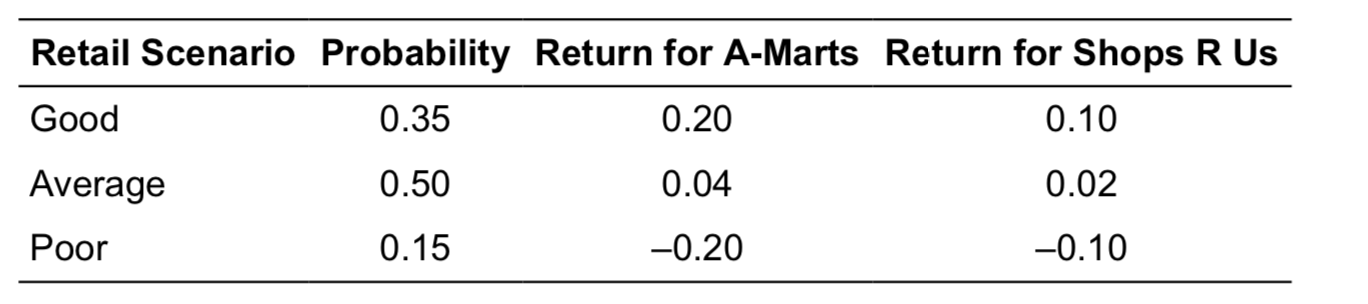Scan QR code or get instant email to install app

Question:

# The joint probability distribution for the return of two retail stocks, A-Marts and Shops R Us, is provided below. The covariance between returns for A-Marts and Shops R Us is:A at least 0, but less than 0.01.
explanation

To calculate the covariance, you first must calculate the expected returns (means) for each stock: Expected return for A-Marts: 0.35(0.20) + 0.50(0.04) + 0.15(–0.20) = 0.06
Expected return for Shops R Us: 0.35(0.10) + 0.50(0.02) + 0.15(–0.10) = 0.03
The covariance is the weighted average of the cross-products:
Covariance = 0.35(0.20 − 0.06)(0.10 − 0.03) + 0.50(0.04 − 0.06)(0.02 − 0.03) + 0.15(–0.20 − 0.06)(–0.10 − 0.03)
Covariance = 0.35(0.14)(0.07) + 0.50(–0.02)(–0.01) + 0.15(–0.26)(–0.13) = 0.0086